Courses

# Karman-Pohlhausen Approximate Method For Solution Of Momentum Integral Equation Over A Flat Plate Mechanical Engineering Notes | EduRev

## Civil Engineering (CE) : Karman-Pohlhausen Approximate Method For Solution Of Momentum Integral Equation Over A Flat Plate Mechanical Engineering Notes | EduRev

The document Karman-Pohlhausen Approximate Method For Solution Of Momentum Integral Equation Over A Flat Plate Mechanical Engineering Notes | EduRev is a part of the Civil Engineering (CE) Course Fluid Mechanics.
All you need of Civil Engineering (CE) at this link: Civil Engineering (CE)

Karman-Pohlhausen Approximate Method For Solution Of Momentum Integral Equation Over A Flat Plate

• The basic equation for this method is obtained by integrating the direction momentum equation (boundary layer momentum equation) with respect to from the wall (at y = 0) to a distance δ(x) which is assumed to be outside the boundary layer. Using this notation, we can rewrite the Karman momentum integral equation as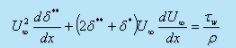(30.1)

• The effect of pressure gradient is described by the second term on the left hand side. For pressure gradient surfaces in external flow or for the developing sections in internal flow, this term contributes to the pressure gradient.
• We assume a velocity profile which is a polynomial of . η = y / δ, η being a form of similarity variable , implies that with the growth of boundary layer as distance varies from the leading edge, the velocity profile u / U remains geometrically similar.
• We choose a velocity profile in the form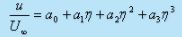(30.2)

In order to determine the constants a0,a1, a2, and  a3 we shall prescribe the following boundary conditions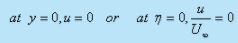(30.3a)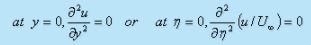(30.3b)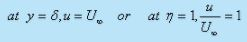(30.3c)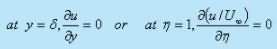(30.3d)

These requirements will yield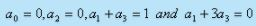respectively
Finally, we obtain the following values for the coefficients in Eq. (30.2),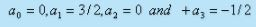and the velocity profile becomes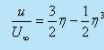(30.4)

For flow over a flat plate,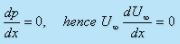and the governing Eq. (30.1) reduces to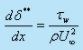(30.5)

Again from Eq. (29.8), the momentum thickness is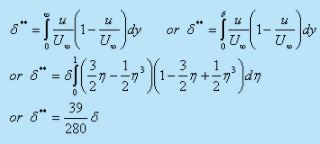The wall shear stress is given by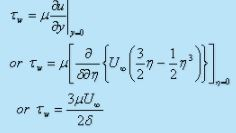• Substituting the values of δ** and τw in Eq. (30.5) we get,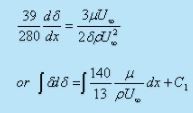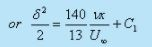(30.6)

where C1 is any arbitrary unknown constant.

• The condition at the leading edge (at x = 0, δ = 0) yields C1 = 0
Finally we obtain,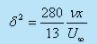(30.7)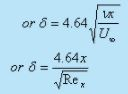(30.8)

• This is the value of boundary layer thickness on a flat plate. Although, the method is an approximate one, the result is found to be reasonably accurate. The value is slightly lower than the exact solution of laminar flow over a flat plate . As such, the accuracy depends on the order of the velocity profile. We could have have used a fourth order polynomial instead --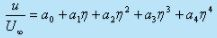(30.9)

• In addition to the boundary conditions in Eq. (30.3), we shall require another boundary condition at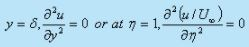• This yields the constants as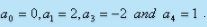. Finally the velocity profile will be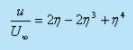• Subsequently, for a fourth order profile the growth of boundary layer is given by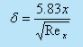(30.10)

Integral Method For Non-Zero Pressure Gradient Flows

• A wide variety of "integral methods" in this category have been discussed by Rosenhead . The Thwaites method  is found to be a very elegant method, which is an extension of the method due to Holstein and Bohlen . We shall discuss the Holstein-Bohlen method in this section
• This is an approximate method for solving boundary layer equations for two-dimensional generalized flow. The integrated  Eq. (29.14) for laminar flow with pressure gradient can be written as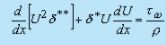or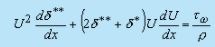(30.11)

• The velocity profile at the boundary layer is considered to be a fourth-order polynomial in terms of the dimensionless distance η = y / δ, and is expressed as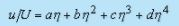The boundary conditions are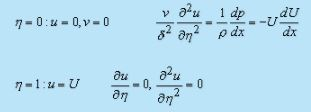• A dimensionless quantity, known as shape factor is introduced as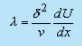(30.12)

• The following relations are obtained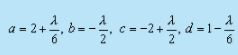• Now, the velocity profile can be expressed as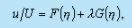(30.13)

where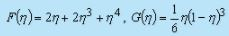• The shear stress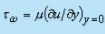is given by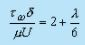(30.14)

• We use the following dimensionless parameters,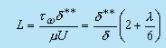(30.15)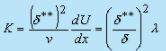(30.16)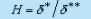(30.17)

• The integrated momentum Eq. (30.10) reduces to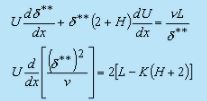(30.18)

• The parameter is related to the skin friction
• If we take as the independent variable and can be shown to be the functions of since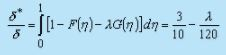(30.19)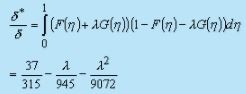(30.20)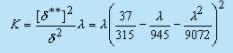(30.21)

Therefore,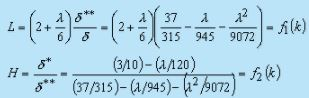• The right-hand side of Eq. (30.18) is thus a function of alone. Walz  pointed out that this function can be approximated with a good degree of accuracy by a linear function of so that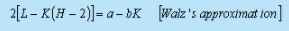• Equation (30.18) can now be written as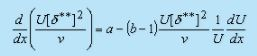Solution of this differential equation for the dependent variable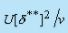subject to the boundary condition  U = 0 when x = 0 , gives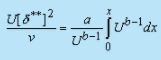• With = 0.47 and = 6. the approximation is particularly close between the stagnation point and the point of maximum velocity.
• Finally the value of the dependent variable is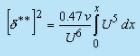(30.22)

• By taking the limit of Eq. (30.22), according to L'Hopital's rule, it can be shown that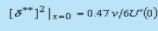This corresponds to K = 0.0783.

• Note that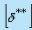is not equal to zero at the stagnation point. If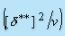is determined from Eq. (30.22),K(x) can be obtained from Eq. (30.16).
• Table 30.1 gives the necessary parameters for obtaining results, such as velocity profile and shear stress τw The approximate method can be applied successfully to a wide range of problems.

Table 30.1    Auxiliary functions after Holstein and Bohlen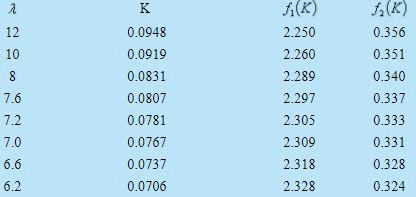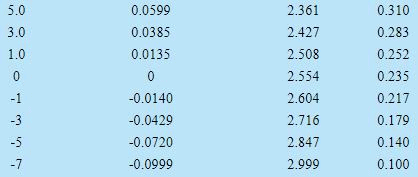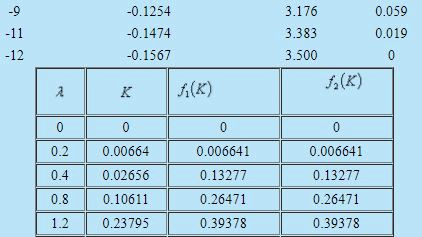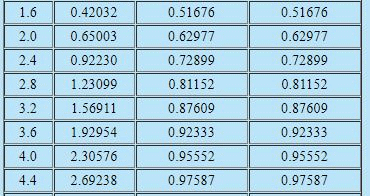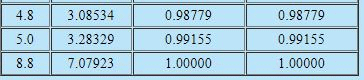• As mentioned earlier, and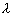are related to the pressure gradient and the shape factor.
• Introduction of andin the integral analysis enables extension of Karman-Pohlhausen method for solving flows over curved geometry. However, the analysis is not valid for the geometries, where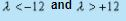Point of Seperation

For point of seperation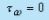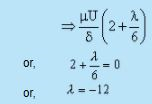Offer running on EduRev: Apply code STAYHOME200 to get INR 200 off on our premium plan EduRev Infinity!

## Fluid Mechanics

43 videos|70 docs|72 tests

,

,

,

,

,

,

,

,

,

,

,

,

,

,

,

,

,

,

,

,

,

;